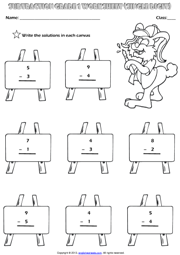Home Maths Main Page

# Single Digit Subtraction Maths Exercise Worksheet

A fun single digit subtraction exercise maths worksheet for grade 1 (first grade) students and kids with rabbit and canvas theme. Write the solutions and colour the rabbit. (Vertical Subtraction Worksheet for kids)Next Worksheet Grade 1 Subtraction Main Page Home